## ABCD Parameters of Two Port Network or Transmission Parameters or Chain Parameters:

ABCD Parameters of Two Port Network are known as transmission parameters. These are generally used in the analysis of power transmission in which the input port is referred as the sending end while the output port is referred as receiving end. These are obtained by expressing voltage V1 and current I1 at input port interms of voltage V2 and current I2 at output port. Thus, voltage V2 and current I2 are independent variables while voltage V1 and current I1 are dependent variables. Thus, we have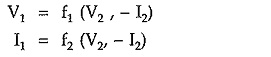Generally we have considered the currents in both the ports are entering the port and both are positive. The negative sign with I2 indicates that, for the ABCD parameters the current I2 is leaving the port 2.

In equation form, above relations can be written as,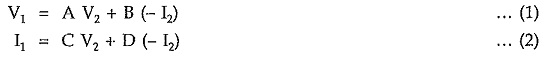In matrix form above equations can be written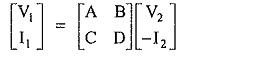The individual transmission parameters are defined as follows,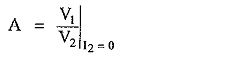This is called open circuit reverse voltage gain.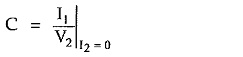This is called open circuit transfer admittance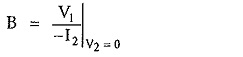This is called short circuit transfer impedance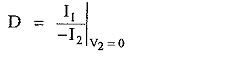This is called short circuit reverse current gain.

ABCD or Transmission parameters are also called chain parameters.

Scroll to Top